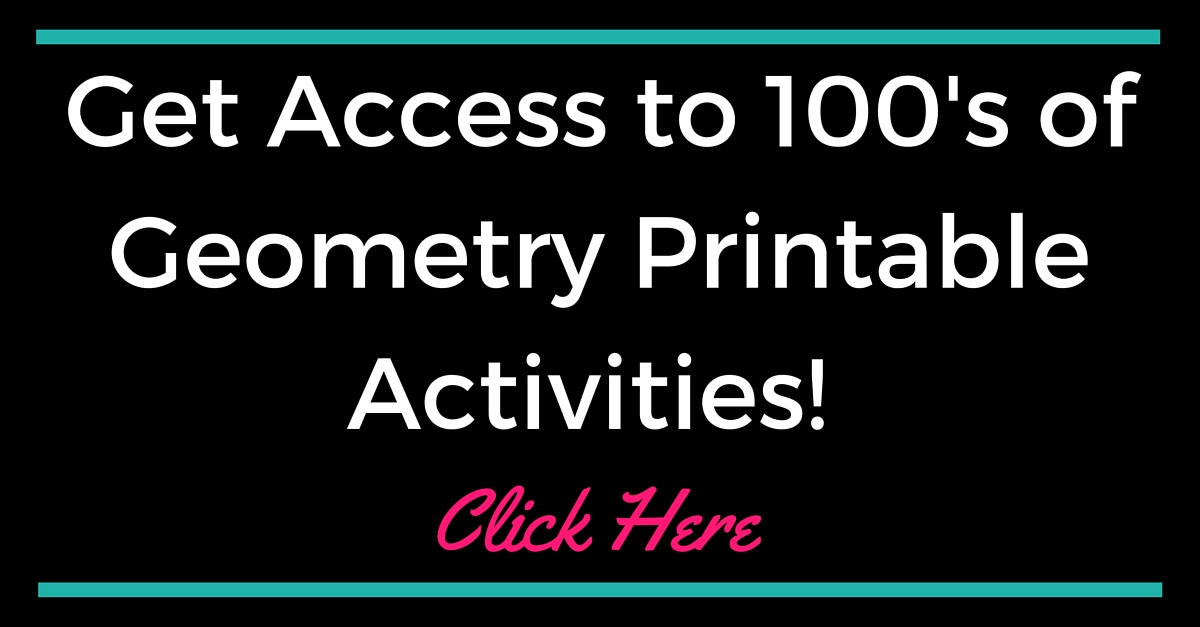# Geometry Curriculum

Geometry Coach - High School Curriculum Map

Here is the Geometry Overview for the Common Core State Standards Initiative
(This information was used to create the Curriculum Map and Lesson Plans along with the Mathematics Common Core Toolbox)

Congruence

• Experiment with transformations in the plane
• Understand congruence in terms of rigid motions
• Prove geometric theorems
• Make geometric constructions

Similarity, Right Triangles, and Trigonometry

• Understand similarity in terms of similarity transformations
• Prove theorems involving similarity
• Define trigonometric ratios and solve problems involving right triangles
• Apply trigonometry to general triangles

Circles

• Understand and apply theorems about circles
• Find arc lengths and areas of sectors of circles

Expressing Geometric Properties with Equations

• Translate between the geometric description and the equation for a conic section
• Use coordinates to prove simple geometric theorems algebraically

Geometric Measurement and Dimension

• Explain volume formulas and use them to solve problems
• Visualize relationships between two-dimensional and three-dimensional objects

Modeling with Geometry

• Apply geometric concepts in modeling situations

Mathematical Practices

1. Make sense of problems and persevere in solving them.
2. Reason abstractly and quantitatively.
3. Construct viable arguments and critique the reasoning of others.
4. Model with mathematics.
5. Use appropriate tools strategically.
6. Attend to precision.
7. Look for and make use of structure.
8. Look for and express regularity in repeated reasoning.